# High School Math : Solving Logarithms

## Example Questions

### Example Question #1 : Exponential And Logarithmic Functions

You are given that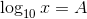and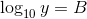Which of the following is equal to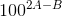?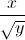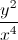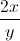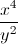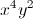Explanation:

Sinceand, it follows that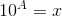and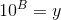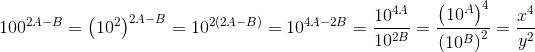### Example Question #1 : Exponential And Logarithmic Functions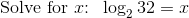Explanation: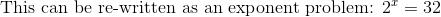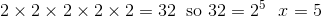### Example Question #3 : Exponential And Logarithmic Functions

What is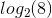Recall that by definition a logarithm is the inverse of the exponential function. Thus, our logarithm corresponds to the value ofin the equation: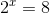We know that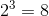and thus our answer is.